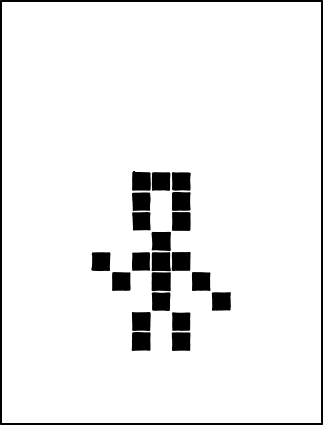## more games of life

Posted in Books, Kids, R with tags , , , , on May 5, 2020 by xi'anAnother puzzle in memoriam of John Conway in The Guardian:

Find the ten digit number, abcdefghij. Each of the digits is different, and

• a is divisible by 1
• ab is divisible by 2
• abc is divisible by 3
• abcd is divisible by 4
• abcde is divisible by 5
• abcdef is divisible by 6
• abcdefg is divisible by 7
• abcdefgh is divisible by 8
• abcdefghi is divisible by 9
• abcdefghij is divisible by 10

Which brute force R coding by checking over random permutations of (1,2,…,9) [since j=0] solves within seconds:

```while(0<1)
if (prod(!(x<-sum(10^{0:8}*sample(1:9)))%/%10^{7:0}%%2:9))break()
```

into x=3816547290. And slightly less brute force R coding even faster:

```while(0<1){
e=sample(c(2,6,8))#even
o=sample(c(1,3,7,9))#odd
if((!(o+e+o)%%3)&
(!(10*o+e)%%4)&
(!(o+e+o+e+5+4)%%3)&
(!sum(10^{6:0}*c(o,e,o,e,5,4,o))%%7)&
(!(10*o+e)%%8)&
(!(sum(o)+sum(e))%%9)){
print(sum(10^{9:0}*c(o,e,o,e,4,5,o,e,o,0)));break()}}
```

## Le Monde puzzle [#1141]

Posted in Kids, pictures, R, University life with tags , , , , , , , , on May 4, 2020 by xi'anThe weekly puzzle from Le Monde is in honour of John Conway, who just passed away, ending up his own game of life:

On an 8×8 checker-board, Alice picks n squares as “infected”. She then propagates the disease by having each square with least two infected neighbours to become infected as well. What is the minimal value of n for the entire board to become infected? What if three infected neighbours are required?

A plain brute force R random search for proper starting points led to n=8 (with a un-code-golfed fairly ugly rendering of the neighbourhood relation, I am afraid!) with the following initial positionWith three neighbours, an similar simulation failed to return anything below n=35 as for instance:oops, n=34 when running a little longer:which makes sense since an upper bound is found by filling one square out of two (32) and adding both empty corners (2). But this upper bound is only considering one step ahead, so is presumably way too large. (And indeed the minimal value is 28, showing that brute force does not always work! Or it may be that forcing the number of live cells to grow at each step is a coding mistake…)

## Le Monde puzzle [#1139]

Posted in Books, Kids, R, Statistics with tags , , , , , on April 24, 2020 by xi'anA weekly Monde current mathematical puzzle that reminded me of an earlier one (but was too lazy to check):

The integer n=36 enjoys the property that all the differences between its ordered divisors are also divisors of 36. Find the only 18≤m≤100 that enjoys this property such that all its prime dividers areof multiplicity one. Are there other such m’s?

The run of a brute force R search return 42 as the solution (codegolf welcomed!)

```y=z=1:1e5
for(x in y)z[y==x]=!sum(x%%diff((1:x)[!x%%(1:x)]))
y=y[z==1]
for(k in generate_primes(2,max(y)))y=y[!!y%%k^2]
```

where generate_primes is a primes R function. Increasing the range of y’s to 10⁵ exhibits one further solution, 1806.

## Le Monde puzzle [#1133]

Posted in Books, Kids, R with tags , , , , , , on March 28, 2020 by xi'anA weekly Monde current mathematical puzzle that reminded me of an earlier one (but was too lazy to check):

If ADULE-ELUDA=POINT, was is the largest possible value of POINT? With the convention that all letters correspond to different digits and no digit can start with 0. Same question when ADULE+ELUDA=POINT.

The run of a brute force R search return 65934 as the solution (codegolf welcomed!)

```dify<-function(adule)
num2dig<-function(dif) (dif%/%10^(0:4))%%10
sl=NULL
for (t in 1:1e6){
sl=as.matrix(distinct(as.data.frame(sl),.keep_all = TRUE))
```

where distinct is a dplyr R function.

```> 94581-18549
 76032
```

The code can be easily turned into solving the second question

```> 31782+28713
 60495
```

## Le Monde puzzle [#1134]

Posted in Books, R with tags , , , , , , on March 24, 2020 by xi'anA Monde mathematical puzzle on gcd’s and scm’s:

If one replaces a pair (a,b) of integers with the pair (g,s) of their greatest common denominator and smallest common multiple, how long at most before the sequence ends. Same question when considering a collection of five integers where two are selected by the pair (g,s) of their greatest common denominator and smallest common multiple.

The first question is straightforward as s is a multiple of s. So the sequence ends at most after one run. For five, run of a brute force R search return 9 as “the” solution (even though the true maximum is 10, as illustrated by the quintuplet (16,24,36,54,81):

```ogcd <- function(x,y){r<-x%%y
return(ifelse(r,ogcd(y,r),y))}

oscm<-function(x,y) x*y/ogcd(x,y)

divemul<-function(a,b) return(c(oscm(a,b),ogcd(a,b)))

for (t in 1:1e5){
ini=sample(1:1e2,5)
i=0;per=ker=sample(ini,2)
nez=divemul(per,per)
while(!max(nez%in%per)){
ini=c(ini[!ini%in%per],nez)
per=sample(ini,2)
ker=rbind(ker,per)
nez=divemul(per,per)
i=i+1}
sol=max(sol,i)}
```